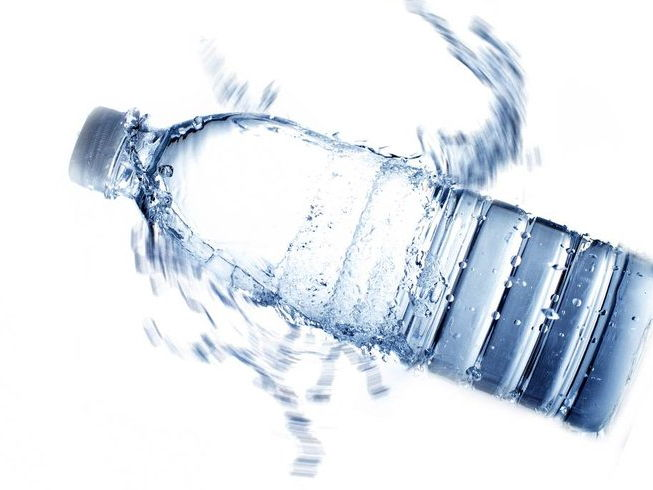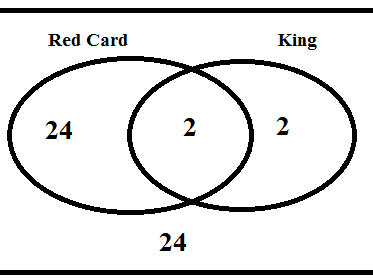## GCSE maths - probability resources

Discover our collection of GCSE maths probability lessons, activities, powerpoints and notes. These resources are hand-picked by the Tes Maths Panel for their relevance to the 9-1 specifications - use them for planning, homework and revision.

This page contains the very best resources for teaching sections P1 to P9 from the GCSE maths specification (first examined in 2017), combining structured lessons and engaging activities. With rules to remember, counter-intuitive results to avoid, and the brand new topic of Venn diagrams, this collection of resources and activities should prove really useful as you plan lessons on probability.

View more Maths topics

## Probability of single events#### KS3 Probability of One Event

KS3 Maths worksheets and activities. The topic of Probability from the Year 7 book of the Mathematics Enhancement Program (MEP – Year 7 – Unit 21). For information about these resources and an index for the whole collection please visit http://www.mrbartonmaths.com/CIMT.htm Keywords: Probability, Chance, Likelihood, Mutually Exclusive, Independent, Impossible, Certain, Relative Frequency, Outcome, Trials, Experiment, Even, Likely, Unlikely, Estimation, Complementary, Addition Law.#### Playing Card Probabilities - KS3 Activity

A KS3 Probability Activity created to help pupils understand the make up of a pack of playing cards (so many didn't know!) and then use this to calculate probabilities - could also be extended further to combined probabilities and tree diagrams etc.#### Probability starter

Multiple choice, intended for use with interactive voting systems. Can be used early on in the teaching of probability to year 7's.#### Intro to Probability and Probability Scale

Level 5 introduction to probability and using the probability scale. Moves on to show how simple probability is calculated using a tube of Smarties. Class should be ready to access your worksheet of probability questions after viewing this. Please rate/comment/favourite if you download. All suggestions to improve are welcome! Dave

## Combined events and sample space diagrams#### Sample Spaces

In this lesson students get shown how a sample space can help when finding combinations of events. They then go onto using the diagram to calculate probabilities based on those events. The worksheet has a variety of difficulties of question and includes answers. If you liked this resource then please check out my other stuff on TES! All free!

https://www.tes.com/teaching-resources/shop/Owen134866#### KS3 Probability Dice Game (using sample spaces)

A nice PowerPoint activity that can be played with KS3 pupils and then they can be left to investigate how probability can be used to determine the best strategy (3 blank faced dice needed).

## Experimental probability and relative frequency#### Probability Race Game with Walkthrough and Extension

These slides show a full and detailed walkthrough of the Probability Race Game.

Students will need a pair of dice and can complete this work as individuals, pairs or small groups.

There are extension activities included and there are worksheets to save time, if required.

Please feel free to feedback any questions, queries or amendments.#### Experimental Probability - Bottle Flipping

I created this PowerPoint as an engaging end of term activity for my low attaining year ten class to investigate the relationship between amount of water in a bottle and the chances of it being flipped successfully. I used the resource to revise experimental probability but the wider investigation covers collecting data, a bit of FDP etc. I know bottle flipping is a bit of a sore point but for some but my class were fully engaged and it helped given them an appreciation of experimental probability. Worksheets are included for groups to complete.#### Three dice simulation on excel. Probability.

Spreadsheet simulating the throwing of three dice and displays a bar chart comparing relative frequency to theoretical probability.

## Tree diagrams - unconditional#### Tree Diagrams

This lesson is intended as an introduction to Tree diagrams. In it, pupils see how Tree diagrams can be used to show different combinations of events, and can therefore be used to calculate probabilities. There are no probabilities on the diagrams at this point, this idea can be introduced at a later date. The worksheet has a variety of questions, with answers included. If you liked this resource then please check out my other stuff on TES - all free!

https://www.tes.com/teaching-resources/shop/Owen134866#### Paper Scissors Stone - Probability Activity - GCSE

A GCSE activity: uses Paper Scissors Stone (or Rock) game to introduce and use probability space diagrams and probability tree diagrams.#### Probability (Tree Diagrams) Codebreaker

This is a poor joke whose punchline that is discovered by solving the probability problems listed. One has a tree diagram drawn and the others could use a tree diagram but ultimately they can be done without.

## Tree diagrams - conditional#### Tree Diagrams - Challenging and Extension Problems

Some questions to delve a little deeper into the understanding of probability and tree diagrams. The first two are fairly standard (I)GCSE fare but subsequent questions become more complex with questions 5 and 6 requiring the solution of quadratic equations if an algebraic approach is used. Suitable for KS4 students who are ready for something a little more challenging than the standard GCSE style question. Answers included.#### Tree diagrams

Tree diagrams including conditional probability- visuals to show how many sweets are left after I've eaten the first one and go back for another.#### Probability conditional without trees lesson

Three part lesson on calculating grade A and grade A* conditional probability without tree diagrams. Task and extension questions provided with fully worked out solutions. Starter recaps previous learning on number topics. Mini-plenary and plenary activities embedded with solutions.

## Venn diagram notation#### Venn Diagrams

2 Worksheets on drawing/reading Venn diagrams with increasing difficulty

## Probability with Venn diagrams#### Venn Diagrams and Probability New GCSE

A lesson on calculating the probability from Venn diagrams (does not include set notation) and designing them from given information.#### Independence, Dependence, Mutually Exclusive or Not?

A worksheet using new GCSE set notation to show independence (using P(A) x P(B)= P(A^B)) and finding probability of one event or another (using P(AUB) = P(A) + P(B) - P(A^B)).
Search by exam board